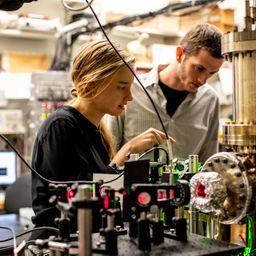## 0.078 \mathrm{nm}

Quantum Physics

Atomic Physics

### Discussion

You must be signed in to discuss.
##### Top Physics 103 Educators##### Jared E.

University of Winnipeg

### Video Transcript

So we're told that potassium iodide has an interplay nurse facing of 0.29 16 nanometers. So D actually not Lambda 0.296 times. 10 to the minus nine meters, 90.29 16 minus nine. And we want to God. We know that the first order Max occurs at 7.6 degrees, and that's the grazing angle, and we want to get the wavelength. So let's just be cautious. Actually, go ahead and write this down, and then I'll start talking. So data is 7.6 degrees, and we this is the grazing angle. So let's go back to the text and figure out what angle does. Um, the data in the brag slow refer to so you actually just roam around 11 more. But, um, we want to use brags law to predict the wavelength. So that's two D signed data is equal to him. Lambda. I wonder how wavelengths for originally measured because, like I think wavelength, we're kind of used to measure spacing and particles. But I wonder how goes the opposite way, Um, at least how it used to in the past. Now I know there's frequency combs, but anyway, Um, so this is our formula. And then I was looking back the diagram. And then this data is in fact, the grazing angle. Um, no, because if you look at figure 27.13 so great. So, um, with this equation, I could say Emma's one, because it's for, say, its first order. So then Lambda is just too d signed data. So if I go ahead and evaluate to decide, they'd ah, see food kind 0.296 time friend of the minus nine. Remember that number right point through 96 ton of minus nine and then times a sign of 7.6 degrees. I got 783 um, 7.83 times, 10 to the minus 11 meters. And is there anything else that this question wants?University of Washington

#### Topics

Quantum Physics

Atomic Physics

##### Top Physics 103 Educators##### Jared E.

University of Winnipeg